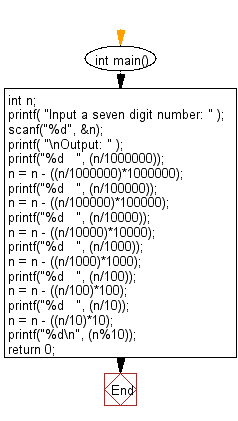﻿ C : Separate one seven-digit number into individual digits

# C Exercises: Accepts one seven-digit number and separates the number into its individual digits, and prints the digits separated from one another by two spaces each

## C Basic Declarations and Expressions: Exercise-75 with Solution

Write a C program that accepts a seven-digit number, separates the number into its individual digits, and prints the digits separated from one another by two spaces each.

Sample Input: 2345678

Sample Solution:

C Code:

``````#include<stdio.h>
int main()
{
int n;

// Prompt for input
printf( "Input a seven digit number: " );

scanf("%d", &n);

printf( "\nOutput: " );

// Extract and print each digit
printf("%d   ", (n/1000000));
n = n - ((n/1000000)*1000000);

printf("%d   ", (n/100000));
n = n - ((n/100000)*100000);

printf("%d   ", (n/10000));
n = n - ((n/10000)*10000);

printf("%d   ", (n/1000));
n = n - ((n/1000)*1000);

printf("%d   ", (n/100));
n = n - ((n/100)*100);

printf("%d   ", (n/10));
n = n - ((n/10)*10);

printf("%d\n", (n%10));

return 0;
}

``````

Sample Output:

```Input a seven digit number:
Output: 2   3   4   5   6   7   8
```

Pictorial Presentation:Flowchart:C programming Code Editor:

What is the difficulty level of this exercise?

Test your Programming skills with w3resource's quiz.

﻿